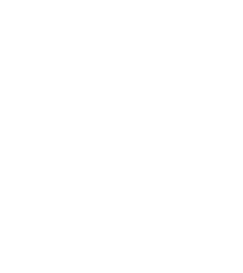Word Drops

How to say "Equation" in Korean.American English

equation

등식

Korean
Ready to learn "Equation" and 16 other words for Mathematics in Korean? Use the illustrations and pronunciations below to get started.
More Mathematics Vocabulary in Korean
Example sentences
American EnglishThe pattern can be summarized by a simple math equation.
Learn the word for "Equation" in 32 More Languages.
Other interesting topics in Korean